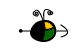Boiling Point Elevation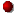Click here to review boiling of pure liquids.

The Macroscopic View

When a solute is added to a solvent, the vapor pressure of the solvent (above the resulting solution) is less than the vapor pressure above the pure solvent. The boiling point of a solution, then, will be greater than the boiling point of the pure solvent because the solution (which has a lower vapor pressure) will need to be heated to a higher temperature in order for the vapor pressure to become equal to the external pressure (i.e., the boiling point).

The boiling point of the solvent above a solution changes as the concentration of the solute in the solution changes (but it does not depend on the identity of either the solvent or the solute(s) particles (kind, size or charge) in the solution).

Non-Volatile Solutes

The boiling point of the solvent above a solution will be greater than the boiling point of the pure solvent whether the solution contains a non-volatile solute or a volatile solute. However, for simplicity, only non-volatile solutes will be considered here.

Experimentally, we know that the change in boiling point of the solvent above a solution from that of the pure solvent is directly proportional to the molal concentration of the solute: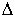T = Kbm

where:T is the change in boiling point of the solvent,
Kb is the molal boiling point elevation constant, and
m is the molal concentration of the solute in the solution.

Note that the molal boiling point elevation constant, Kb, has a specific value depending on the identity of the solvent.

 solvent normal boiling point, oC Kb, oC m-1 water 100.0 0.512 acetic acid 118.1 3.07 benzene 80.1 2.53 chloroform 61.3 3.63 nitrobenzene 210.9 5.24

The following graph shows the normal boiling point for water (solvent) as a function of molality in several solutions containing sucrose (a non-volatile solute). Note that the normal boiling point of water increases as the concentration of sucrose increases.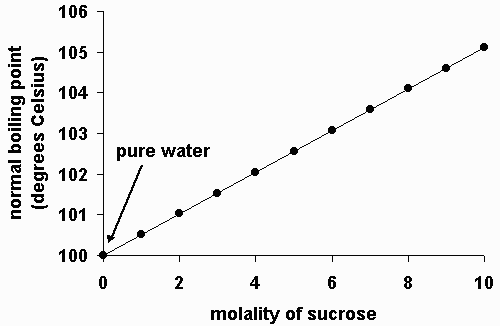The Microscopic View

The figure below shows a microscopic view of the surface of pure water. Note the interface between liquid water (below) and water vapor (above).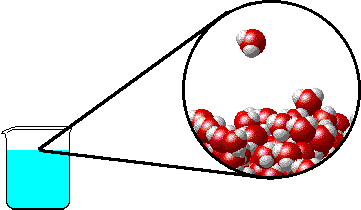Non-Volatile Solutes

The figures below illustrate how the vapor pressure of water is affected by the addition of the non-volatile solute, NaCl.

Note that:

• there are fewer water molecules in the vapor (i.e., lower vapor pressure) above the NaCl solution than in the vapor above pure water, and
• the boiling point of the NaCl solution will be greater than the boiling point of pure water.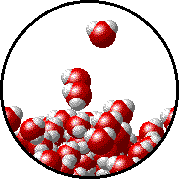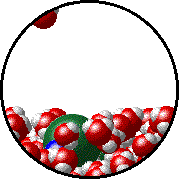Pure water - microscopic view.Normal boiling point = 100.0oC. 1.0 M NaCl solution - microscopic view.Normal boiling point = 101.0oC. Note that the ionic solid, NaCl, produces Na+ ions (blue) and Cl- ions (green) when dissolved in water.